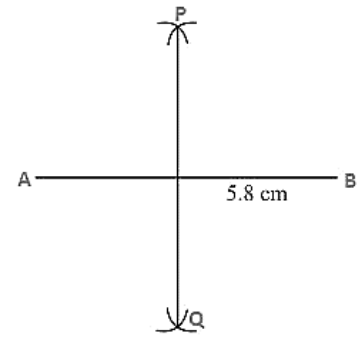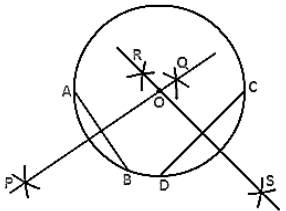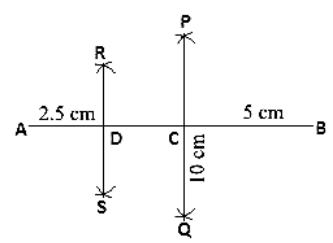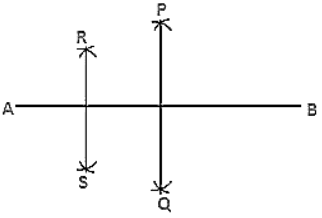Click to Chat

1800-1023-196

+91-120-4616500

CART 0

• 0

MY CART (5)

Use Coupon: CART20 and get 20% off on all online Study Material

ITEM
DETAILS
MRP
DISCOUNT
FINAL PRICE
Total Price: Rs.

There are no items in this cart.
Continue Shopping• Complete JEE Main/Advanced Course and Test Series
• OFFERED PRICE: Rs. 15,900
• View Details

```Chapter 17: Constructions Exercise – 17.1

Question: 1

Draw a line segment of length 8.6 cm. Bisect it and measure the length of each part.

Solution:Steps of construction:

1. Draw a line segment AB of 8.6 cm.

2. Keeping A as center and radius more than half of AB draw arcs on each side of AB.

3. Keeping B as center and the same radius draw arcs on each side of AB cutting the previous arcs at P and Q respectively.

4. Join the points P and Q which intersects AB at C.

Therefore AC = BC = 4.3 cm

Question: 2

Draw a line segment AB of length 5.8 cm. Draw the perpendicular bisector of this line segment.

Solution:Steps of construction:

1. Draw a line segment AB of 5.8 cm.

2. Keeping A as center and radius more than half of AB draw arcs on each side of AB.

3. Keeping B as center and the same radius draw arcs on each side of AB cutting the previous arcs at P and Q respectively.

4. Join the points P and Q.

Hence PQ is the perpendicular bisector of AB

Question: 3

Draw a circle with center at point O and radius 5 cm. Draw its chord AB, the perpendicular bisector of line segment AB. Does it pass through the center of the circle?

Solution:Steps of construction:

1. Keeping O as center and radius as 5 cm draw a circle.

2. Draw a chord AB.

3. Keeping A as center and radius more than half of AB draw arcs one on each side of chord AB.

4. Keeping B as center and the same radius draw arcs cutting the previous arcs at points P and Q respectively.

5. Join the points P and Q.

Therefore the perpendicular bisector PQ of chord AB passes through the center of the circle.

Question: 4

Draw a circle with center at point O. Draw its two chords AB and CD such that AB is not parallel to CD. Draw the perpendicular bisectors of AB and CD. At what point do they intersect?

Solution:Steps of construction:

1. Keeping O as the centre and any radius draw a circle.

2. Draw two chords AB and CD.

3. Keeping A as center and radius more than half of AB draw arcs, one on each side of AB.

4. Keeping B as center and the same radius draw arcs cutting the previous arcs at point P and Q respectively.

5. Join the points P and Q.

6. Keeping D as center and radius more than half of DC draw arcs, one on each side of DC.

7. Keeping C as center and the same radius draw arcs cutting the previous arcs at points R and S respectively.

8. Join the points R and S.

Both the perpendicular bisectors PQ and RS intersect each other at the center O of the circle.

Question: 5

Draw a line segment of length 10 cm and bisect it. Further, bisect one of the equal parts and measure its length.

Solution:Steps of construction:

1. Draw a line segment AB of length 10 cm.

2. Keeping A as center and radius more than half of AB draw arcs one on each side of AB.

3. Keeping B as center and same radius draw arcs cutting the previous arc at point P and Q respectively.

4. Join P and Q which intersects AB at C.

5. Keeping A as center and radius more than half of AC draw arcs on each side of AC.

6. Keeping C as center and the same radius draw arcs cutting the previous arcs at points R and S respectively.

7. Join points R and S which intersects AC at D.

Question: 6

Draw a line segment AB bisect it. Bisect one of the equal parts to obtain a line segment of length (1/4) (AB).

Solution:Steps of construction:

1. Draw a line segment AB.

2. With A as center and radius more than half of AB draw arcs, one on each side of AB.

3. With B as the center and same radius draw arcs cutting the previous arcs at points P and Q respectively.

4. Join P and Q which intersects AB at C.

5. With A as center and radius more than half of AC draw arcs, one on each side of AC.

6. With C as the center and same radius draw arcs cutting the previous arcs at R and S respectively.

7. Join points R and S which intersects AC at D.

Question: 7

Draw a line segment AB and by ruler and compasses, obtain a line segment of length (3/4)(AB).

Solution:Steps of construction:

1. Draw a line segment AB.

2. With A as center and radius more than half of AB draw arcs, one on each side of AB.

3. With B as the center and same radius draw arcs cutting the previous arcs at points P and Q respectively.

4. Join P and Q which intersects AB at C.

5. With A as center and radius more than half of AC draw arcs, one on each side of AC.

6. With C as the center and same radius draw arcs cutting the previous arcs at R and S respectively.

7. Join points R and S which intersects AC at D.

```### Course Features

• 728 Video Lectures
• Revision Notes
• Previous Year Papers
• Mind Map
• Study Planner
• NCERT Solutions
• Discussion Forum
• Test paper with Video Solution